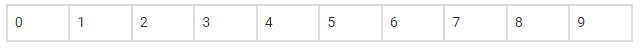# How many automobile license plates`
Question:

How many automobile license plates can be made if each plate contains two different letters followed by three different digits?

Solution:

According to the question,

Number of letters in automobile license plates = 2

We know that,

There are 26 alphabets

So, Letter can be arranged without repetition in the following number of ways,

= 26×25

=650

Number of digits in automobile license plates =3

We know that, there 10 digitsHence the number of digits without repetitions

=10×9×8=720

Therefore, the total number of way automobile license plates

=720×650

=468000